Home and Learn: Microsoft Excel Course

# Excel Goal Seek

Goal Seek is used to get a particular result when you're not too sure of the starting value. For example, if the answer is 56, and the first number is 8, what is the second number? Is it 8 multiplied by 7, or 8 multiplied by 6? You can use Goal Seek to find out. We'll try that example to get you started, and then have a go at a more practical example.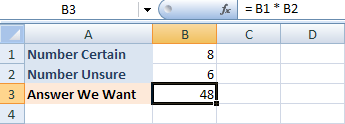In the spreadsheet above, we know that we want to multiply the number in B1 by the number in B2. The number in cell B2 is the one we're not too sure of. The answer is going in cell B3. Our answer is wrong at the moment, because we have a Goal of 56. To use Goal Seek to get the answer, try the following:

• From the Excel menu bar, click on Data
• Locate the Data Tools panel and the What if Analysis item. (In Excel 2016, the What If item is on the Forecast panel, next to Data Tools.) From the What if Analysis menu, select Goal Seek
• The following dialogue box appears: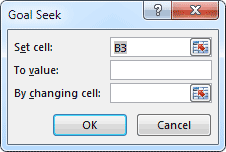The first thing Excel is looking for is "Set cell". This is not very well named. It means "Which cell contains the Formula that you want Excel to use". For us, this is cell B3. We have the following formula in B3:

= B1 * B2

So enter B3 into the "Set cell" box, if it's not already in there.

The "To value" box means "What answer are you looking for"? For us, this is 56. So just type 56 into the "To value" box

The "By Changing Cell" is the part you're not sure of. Excel will be changing this part. For us, it was cell B2. We're weren't sure which number, when multiplied by 8, gave the answer 56. So type B2 into the box.

You Goal Seek dialogue box should look like ours below: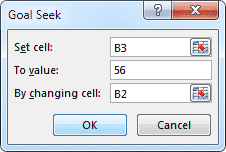Click OK and Excel will tell you if it has found a solution: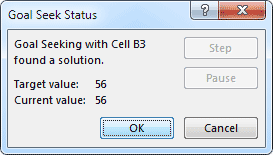Click OK again, because Excel has found the answer. Your new spreadsheet will look like this one:As you can see, Excel has changed cell B2 and replace the 6 with a 7 - the correct answer.

We'll now try a more practical example.

### Goal Seek Number Two

Consider this problem:

Your business has a modest profit of 25,000. You've set yourself a new profit Goal of 35,000. At the moment, you're selling 1000 items at 25 each. Assume that you'll still sell 1000 items. The question is, to hit your new profit of 35,000, by how much do you have to raise your prices?

Create the spreadsheet below, and we'll find a solution with Goal Seek.The spreadsheet is split into two: Current Sales, and Future Sales. We'll be changing the Future Sales with Goal Seek. But for now, enter the same values for both sections. The formula to enter for B4 is this:

= B2 * B3

And the formula to enter for E4 is this:

= E2 * E3

The current Price Per Item is 25.00. We want to change this with Goal Seek, because our prices will be going up to hit our new profits of 35,000. So try this:

• From the Excel menu bar, click on Data
• Locate the Data Tools panel and the What if Analysis item. From the What if Analysis menu, select Goal Seek
• The following dialogue box appears: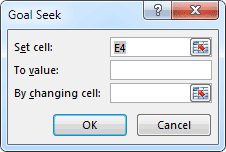For "Set cell", enter E4. This is where the formula is. The "To Value" is what we want our new profits to be. So enter 35000. The "By changing cell" is the part we're not sure of. For us, this was the price each item needs to be increased by. This was coming from cell E3 on our spreadsheet. So enter E3 in the "By changing cell" box. Your Goal Seek dialogue box should now look like this:Click OK to see if Excel can find an answer:Excel is now telling that it has indeed found a solution. Click OK to see the new version of the spreadsheet: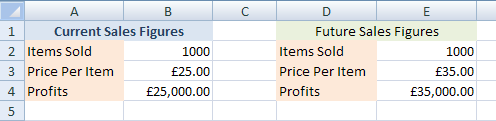Our new Price Per Item is 35. Excel has also changed the Profits cell to 35 000.

Exercise
You've had a meeting with your staff, and it has been decide that a price change from 25 to 35 is not a good idea. A better idea is to sell more items. You still want a profit of 35 000. Use Goal Seek to find out how many items you'll have to sell to meet your new profit figure.

In the next part, well take a closer look at cell references in Excel.

<--Back to the Excel Contents Page

Email us: enquiry at homeandlearn.co.uk